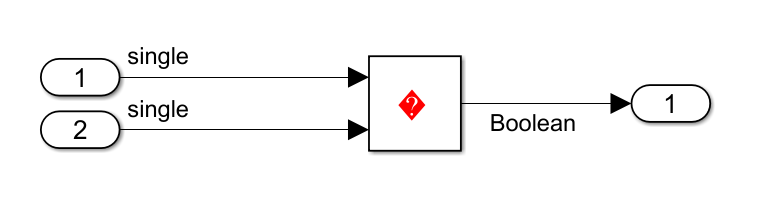Main Content

## Casting modes

### Description

Specify how the code generator casts data types for variables.

Category: Code Generation > Code Style

### Settings

Default: `Nominal`

Nominal

Generate code that uses default C compiler data type casting.

For example:

```void rtwdemo_rtwecintro_step(void) { boolean_T rtb_equal_to_count; rtDWork.X++; rtb_equal_to_count = (rtDWork.X != 16); if (rtb_equal_to_count && (rtPrevZCSigState.Amplifier_Trig_ZCE != POS_ZCSIG)) { rtY.Output = rtU.Input << 1; }```

Standards Compliant

Generate code where data type casting complies with MISRA® standards.

For example:

```void rtwdemo_rtwecintro_step(void) { boolean_T rtb_equal_to_count; rtDWork.X = (uint8_T)((uint32_T)rtDWork.X + 1U); rtb_equal_to_count = ((int32_T)rtDWork.X != 16); if (rtb_equal_to_count && ((uint32_T)rtPrevZCSigState.Amplifier_Trig_ZCE != POS_ZCSIG)) { rtY.Output = rtU.Input << 1U; } ```

Note

The expression `rtY.Output = rtU.Input << 1U` is not compliant with MISRA C:12 Rule 10.1 because the model configuration parameter Replace multiplications by powers of two with signed bitwise shift is selected. For more information, see Replace multiplications by powers of two with signed bitwise shifts.

Depending on the setting, the configuration parameter Casting modes can replace bitwise XOR operations with relational operations in the generated code to satisfy the MISRA C:12 Rule 10.1 when the operands are signed types. For example, generate code from the following model with the Casting modes set to `Nominal` and ```Standard compliant``` respectively.```// Model step function (casting mode set to Nominal) void step(void) {rtY.Out3 = (boolean_T)((int32_T)(rtU.In1 != 0.0F) ^ (int32_T)(rtU.Inport1 != 0.0F)); } // Model step function (Casting modes set to Standard Compliant) void step(void) { rtY.Out3 = ((rtU.In1 != 0.0F) != (rtU.Inport1 != 0.0F)); }```
Here, the parameters `rtU.In1` and `rtU.Inport1` are single singed types. Performing a bitwise XOR(^) operation on these operands violates the MISRA C:12 Rule 10.1. To prevent this violation, the code generator replaces the bitwise XOR(^) operation with an inequality(!=) in the generated code when Casting modes is set to ```Standard compliant```.

Explicit

Generate code that casts data type values explicitly.

For example:

```void rtwdemo_rtwecintro_step(void) { boolean_T rtb_equal_to_count; rtDWork.X = (uint8_T)((uint32_T)(int32_T)rtDWork.X + 1U); rtb_equal_to_count = (boolean_T)((int32_T)rtDWork.X != 16); if (((int32_T)rtb_equal_to_count) && ((int32_T) rtPrevZCSigState.Amplifier_Trig_ZCE != (int32_T)POS_ZCSIG)) { rtY.Output = rtU.Input << 1; } ```

### Command-Line Information

 Parameter: `CastingMode` Type: character vector Value: `'Nominal'` | `'Standards'` | `'Explicit'` Default: `'Nominal'`

### Recommended Settings

ApplicationSetting
DebuggingNo impact
TraceabilityNo impact
EfficiencyNo impact
Safety precautionNo impact

Download guide